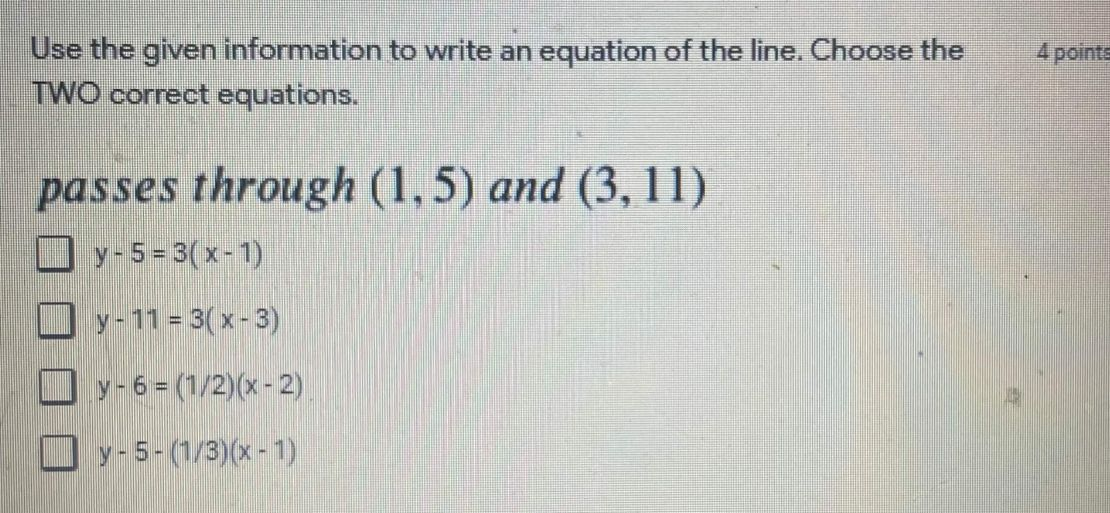### Still have math questions?

Algebra
QuestionUse the given information to write an equation of the line. Choose the TWO correct equations. passes through $$( 1,5 )$$ and $$( 3,11 )$$

$$y - 5 = 3 ( x - 1 )$$

$$y - 11 = 3 ( x - 3 )$$

$$y - 6 = ( 1 / 2 ) ( x - 2 )$$

$$y - 5 - ( 1 / 3 ) ( x - 1 )$$

$$y - 5 = 3 ( x - 1 )$$
$$y - 11 = 3 ( x - 3 )$$